#### 3.1.3Discretization

The basics of Galerkin’s method consist of a clever discretization of the infinite linear space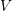in the problem (3.5). The purpose of such a process is to create a controlled sub-space inside, which can be designed to approximate the solution of (3.6) with arbitrary precision.

Consider the finite sub-space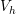ofwith dimension N. Consider also the functions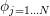as an orthogonal basis of. Hence, every functionincan be written as linear combination of the basis as in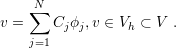(3.9)

The discrete formulation of the variational problem (3.5) can be written as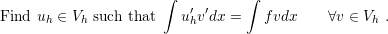(3.10)

Actually, the formulation (3.10) can be simplified. It can be proven that if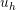satisfies (3.10) only for the basis function of, it satisfies it for every element in. To show this, replacein (3.10) by the basis projection (3.9) as in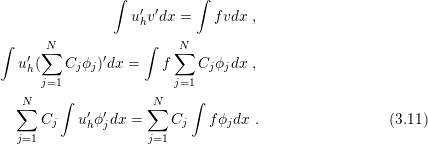On the assumption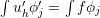for j = 1..N, the equality (3.11) holds andsatisfies (3.10) for every element of. Consequently, the simplified version of (3.10) can be summarized by: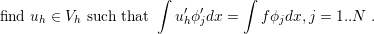(3.12)

The formulation (3.12) is well suitable for the construction of a numerical scheme, because it restricts the search for the solutionto the computation of a finite number of equations (N). Hence, the problem shifts to a more concrete perspective, and a method is required to computefrom those N equations. For that purpose, consider the expansion ofin the basis of, in the same way as was done before for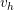(3.9). By substitutingappropriately in (3.10) the obtained function is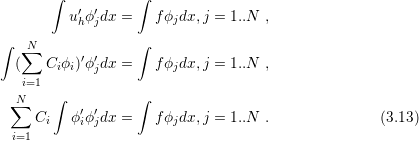In fact, the substitution ofexpansion in (3.12) leads to the transformation of the discrete problem in a linear system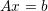with N equations, where A, x and b are given by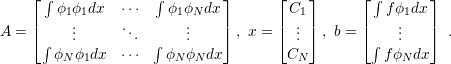(3.14)